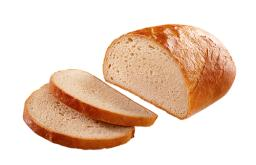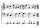They brought in d two kilos and t three kilos of bread to the store. By the end of the day, 14 two-kg bread were sold. How many kilos of bread remained in the store by the next day?

Result

x = kg (Correct answer is: 2d+3t - 14*2)#### Solution:Our examples were largely sent or created by pupils and students themselves. Therefore, we would be pleased if you could send us any errors you found, spelling mistakes, or rephasing the example. Thank you!

Please write to us with your comment on the math problem or ask something. Thank you for helping each other - students, teachers, parents, and problem authors.Tips to related online calculators
Do you have a linear equation or system of equations and looking for its solution? Or do you have quadratic equation?

## Next similar math problems:

• The number 3Ski organizers should print the start numbers from 1 to 45. How many times will they use the number 3 when printing?
• Forest nurseryIn the forest nursery after winter, they found that 1/10 stems died out of them. For them, they land 193 new spruces. How many spruces are in the forest nursery?
• Number of songsWrite an expression for the number of songs they need for this show. Evan and Peter have a radio show that has 2 parts. They need 4 fewer than 11 songs in the first part. In the second part, they need 5 fewer than 3 times the number of songs in the first
• Roman numeralsWrite numbers written in Roman numerals as decimal.
• Seven timesWhich number seven times is just as higher as 27, how much is smaller than 29?
• I thinkI think a number. When I multiply it by five, and after that I subtract 477, I get the same number as if I multiplied it twice. What number do I think?Added together and write as decimal number: LXVII + MLXIV
• Number unknownAdela thought the two-digit number, she added it to its ten times and got 407. What number does she think?
• Equation with one variableSolve the following equation with one unknown: 5(7s + 5) =130
• Write decimalsWrite in the decimal system the short and advanced form of these numbers: a) four thousand seventy-nine b) five hundred and one thousand six hundred and ten c) nine million twenty-six
• ExpressionSolve for a specified variable: P=a+4b+3c, for a
• Simple equation 1035= 7*3*x what is x?
• Six te 2If 3t-7=5t, then 6t=
• Eq1Solve equation: 4(a-3)=3(2a-5)
• Simple equation 8Solve the following equation: 36=-(1+7x)-6(-7-x)
• SimplifySimplify the following problem and express as a decimal: 5.68-[5-(2.69+5.65-3.89) /0.5]
• If-then equationIf 5x - 17 = -x + 7, then x =